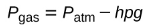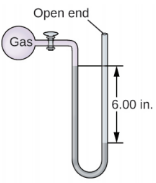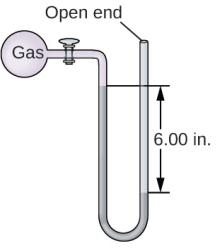# Problem: The pressure of a sample of gas is measured with an open-end manometer, partially shown to the right. The liquid in the manometer is mercury. Assuming atmospheric pressure is 29.92 in. Hg, determine the pressure of the gas in:(b) Pa

###### FREE Expert Solution

For an open-end manometer that has the liquid level lower on the open-ended side, we use the equation below:Where:
Pgas = pressure of the gas
Patm = atmospheric pressure
h= difference in liquid level height
p = density
g = acceleration due to gravity

82% (261 ratings)###### Problem Details

The pressure of a sample of gas is measured with an open-end manometer, partially shown to the right. The liquid in the manometer is mercury. Assuming atmospheric pressure is 29.92 in. Hg, determine the pressure of the gas in:

(b) Pa